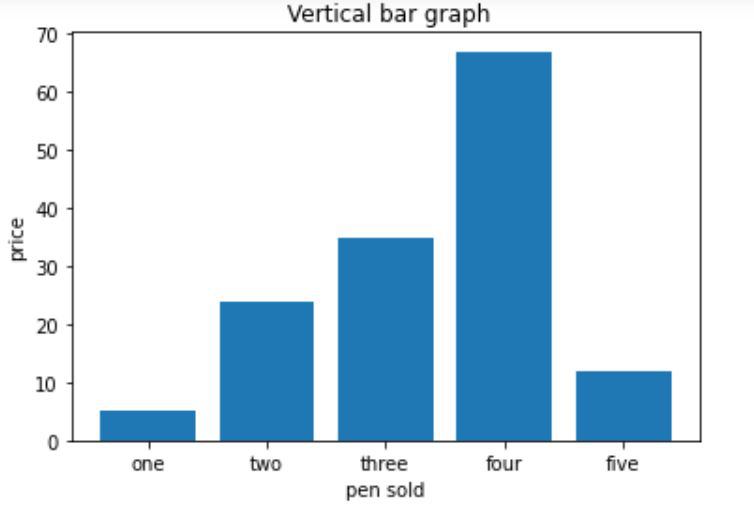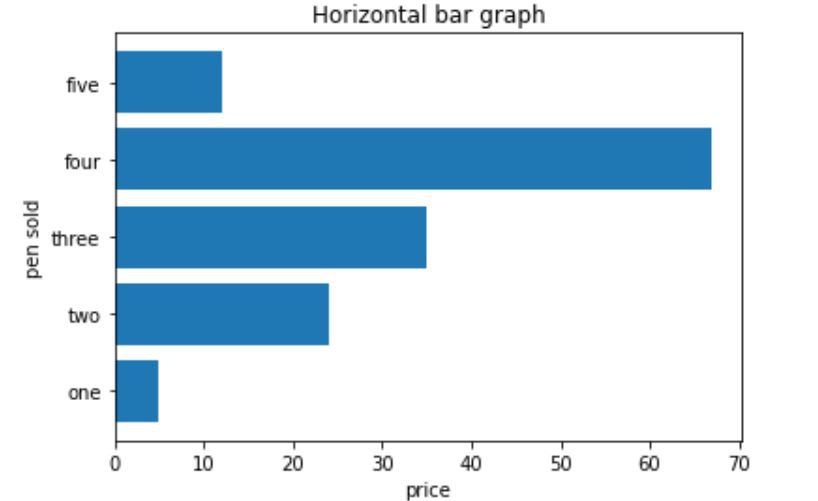# Draw a horizontal bar chart with Matplotlib

Matplotlib is the standard python library for creating visualizations in Python. Pyplot is a module of Matplotlib library which is used to plot graphs and charts and also make changes in them. In this article, we are going to see how to draw a horizontal bar chart with Matplotlib.

### Creating a vertical bar chart

Approach:

• Importing matplotlib.pyplot as plt
• Creating list x for discrete values on x-axis
• Creating list y  consisting only numeric data for discrete values on y-axis
• Calling plt.bar() function with parameters x,y as plt.bar(x,y)
• Setting x_label() and y_label()
• Setting title() for our bar chart
• Calling plt.show() for visualizing our chart

Below is the implementation:

## Python3

 `import` `matplotlib.pyplot as plt`   `x``=``[``'one'``, ``'two'``, ``'three'``, ``'four'``, ``'five'``]`   `# giving the values against` `# each value at x axis` `y``=``[``5``, ``24``, ``35``, ``67``, ``12``] ` `plt.bar(x, y)`   `# setting x-label as pen sold` `plt.xlabel(``"pen sold"``) `   `# setting y_label as price` `plt.ylabel(``"price"``)   ` `plt.title(``" Vertical bar graph"``)` `plt.show()`

Output:### Creating a horizontal bar chart

Approach:

• Importing matplotlib.pyplot as plt
• Creating list y   for discrete values on y-axis
• Creating list x  consisting only numeric data for discrete values on x-axis
• Calling plt.barh() function with parameters y,x as plt.barh(y,x)
• Setting x_label() and y_label()
• Setting title() for our bar chart
• Calling plt.show() for visualizing our chart

Below is the implementation:

## Python3

 `import` `matplotlib.pyplot as plt` `y``=``[``'one'``, ``'two'``, ``'three'``, ``'four'``, ``'five'``]`   `# getting values against each value of y` `x``=``[``5``,``24``,``35``,``67``,``12``] ` `plt.barh(y, x)`   `# setting label of y-axis` `plt.ylabel(``"pen sold"``)`   `# setting label of x-axis` `plt.xlabel(``"price"``) ` `plt.title(``"Horizontal bar graph"``)` `plt.show()`

Output:Whether you're preparing for your first job interview or aiming to upskill in this ever-evolving tech landscape, GeeksforGeeks Courses are your key to success. We provide top-quality content at affordable prices, all geared towards accelerating your growth in a time-bound manner. Join the millions we've already empowered, and we're here to do the same for you. Don't miss out - check it out now!

Previous
Next